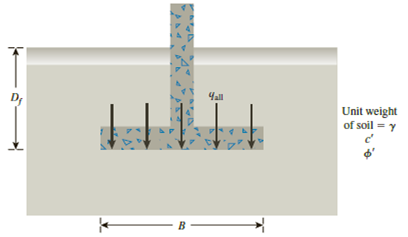Chapter 16, Problem 16.7PPrinciples of Geotechnical Enginee...

9th Edition
Braja M. Das + 1 other
ISBN: 9781305970939

Solutions

Chapter
SectionPrinciples of Geotechnical Enginee...

9th Edition
Braja M. Das + 1 other
ISBN: 9781305970939
Textbook Problem

Redo Problem 16.3 using the modified general ultimate bearing capacity Eq. (16.31).16.1 A continuous footing is shown in Figure 16.17. Using Terzaghi’s bearing capacity factors, determine the gross allowable load per unit area (qall) that the footing can carry. Assume general shear failure. Given: γ = 19 kN/m3, c′ = 31kN/m2, ϕ ′ = 28 ° , Df = 1.5 m, B = 2 m, and factor of safety = 3.5.Figure 16.1716.3 Redo Problem 16.1 with the following: γ = 115 lb/ft3, c′ = 1100 lb/ft2, ϕ ′ = 35 ° , Df = 3.5 ft, B = 5 ft, and factor of safety = 4.

To determine

The gross allowable load per unit area (qall) that the footing can carry using modified general ultimate bearing capacity.

Explanation

Given information:

The unit weight of the soil γ is 115lb/ft3.

The value of cohesion c is 1,100lb/ft2.

The soil friction angle ϕ is 35°.

The depth of foundation Df is 3.5 ft.

The width of footing B is 5 ft.

The factor of safety Fs is 4.0.

Calculation:

Determine the depth factor λcd using the relation.

λcd=1+0.4(DfB)

Substitute 3.5 ft for Df and 5 ft for B.

λcd=1+0.4(3.55)=1.28

Determine the depth factor λqd using the relation.

λqd=1+2tanϕ(1sinϕ)2(DfB)

Substitute 35° for ϕ, 3.5 ft for Df, and 5 ft for B.

λqd=1+2tan35°(1sin35°)2(3.55)=1.178

Determine the ultimate bearing capacity of the soil (qu) using the relation.

qu=cNcλcsλcdλci+qNqλqsλqdλqi+12γBNγλγsλγdλγi=cNcλcsλcdλci+γDfNqλqsλqdλqi+12γBNγλγsλγdλγi (1)

Here, λcs,λqs,andλγs are the shape factors, Nc is the contribution of cohesion, λci,λqi,andλγi are the inclination factors, Nq is the contribution of surcharge, and Nγ is the contribution of unit weight of soil

Still sussing out bartleby?

Check out a sample textbook solution.

See a sample solution

The Solution to Your Study Problems

Bartleby provides explanations to thousands of textbook problems written by our experts, many with advanced degrees!

Get Started

Find more solutions based on key concepts